# SCIENTIFIC NOTATION SCIENTIFIC NOTATIONBASICS Scientific Notation is an

• Slides: 9SCIENTIFIC NOTATIONSCIENTIFIC NOTATIONBASICS - Scientific Notation is an easier way of writing very large or very small numbers Example: The amount of particles in 1 mole of atoms is 60200000000000 Example: The mass of a proton is 0. 00000000000001673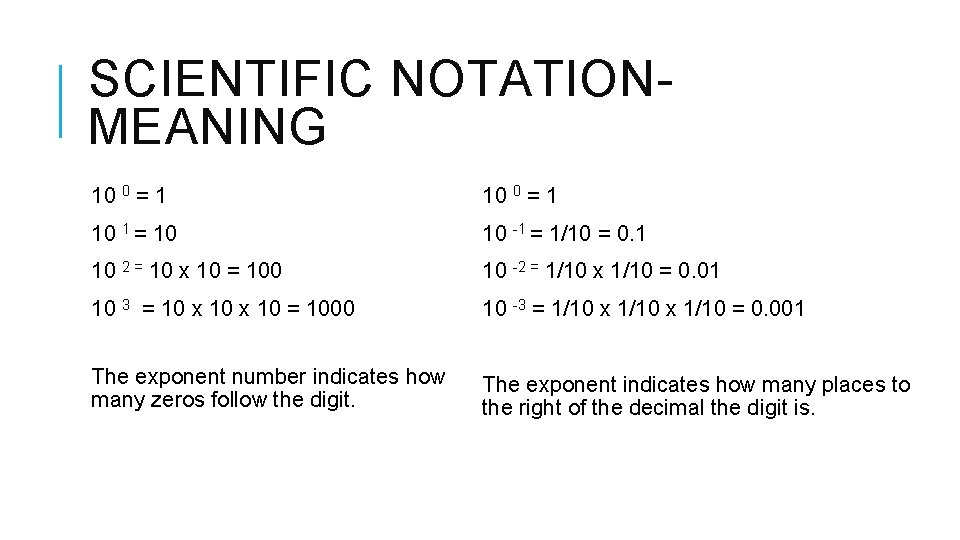SCIENTIFIC NOTATIONMEANING 10 0 = 1 10 1 = 10 10 -1 = 1/10 = 0. 1 10 2 = 10 x 10 = 100 10 -2 = 1/10 x 1/10 = 0. 01 10 3 = 10 x 10 = 1000 10 -3 = 1/10 x 1/10 = 0. 001 The exponent number indicates how many zeros follow the digit. The exponent indicates how many places to the right of the decimal the digit is.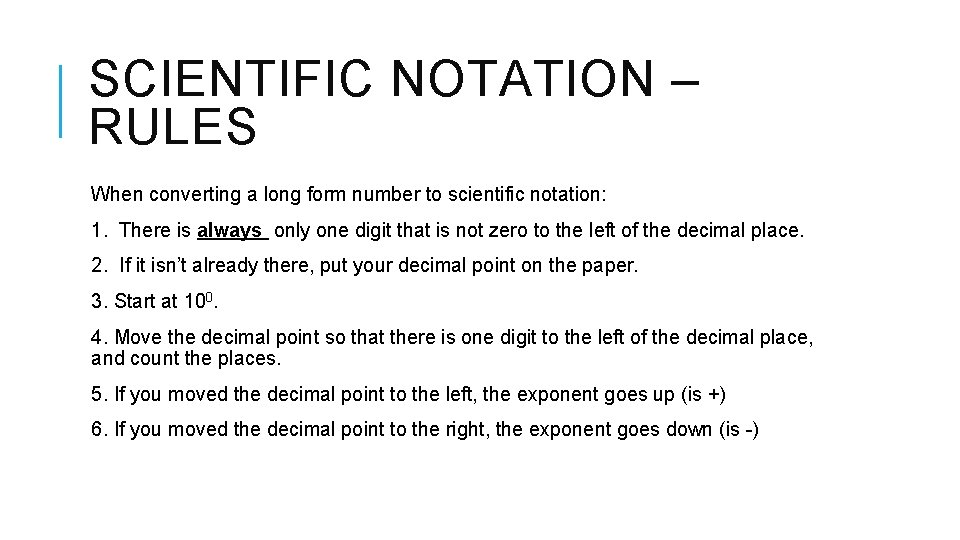SCIENTIFIC NOTATION – RULES When converting a long form number to scientific notation: 1. There is always only one digit that is not zero to the left of the decimal place. 2. If it isn’t already there, put your decimal point on the paper. 3. Start at 100. 4. Move the decimal point so that there is one digit to the left of the decimal place, and count the places. 5. If you moved the decimal point to the left, the exponent goes up (is +) 6. If you moved the decimal point to the right, the exponent goes down (is -)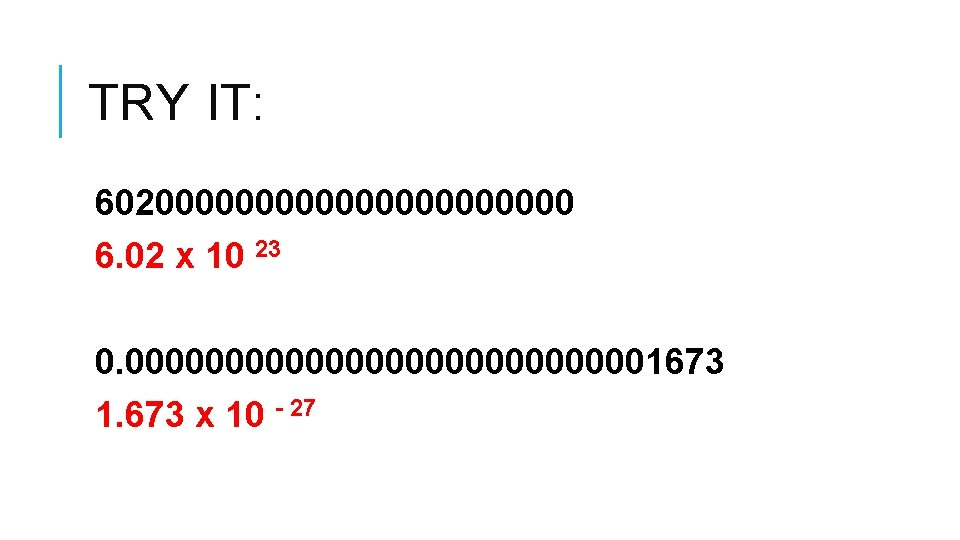TRY IT: 60200000000000 6. 02 x 10 23 0. 00000000000001673 1. 673 x 10 - 27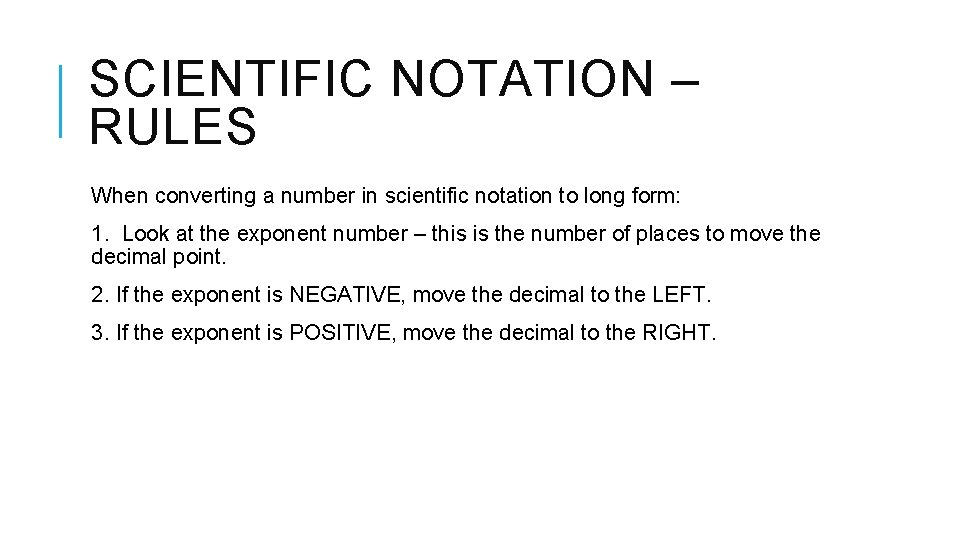SCIENTIFIC NOTATION – RULES When converting a number in scientific notation to long form: 1. Look at the exponent number – this is the number of places to move the decimal point. 2. If the exponent is NEGATIVE, move the decimal to the LEFT. 3. If the exponent is POSITIVE, move the decimal to the RIGHT.TRY IT: 2. 5 x 10 4 25, 000 1. 9 x 10 -5 0. 000019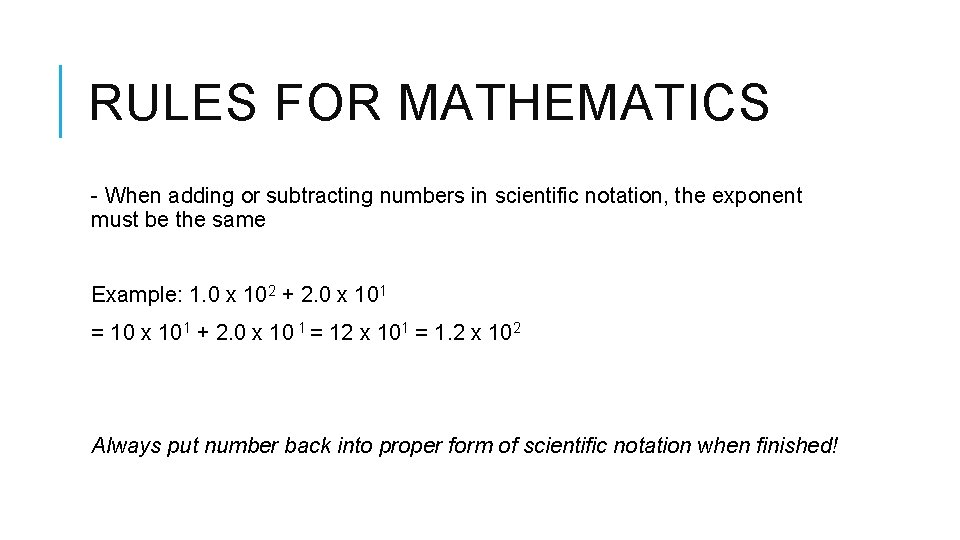RULES FOR MATHEMATICS - When adding or subtracting numbers in scientific notation, the exponent must be the same Example: 1. 0 x 102 + 2. 0 x 101 = 10 x 101 + 2. 0 x 10 1 = 12 x 101 = 1. 2 x 102 Always put number back into proper form of scientific notation when finished!RULES FOR MATHEMATICS - When multiplying numbers in scientific notation, add the exponent Example: (2. 0 x 101)(3. 0 x 102) = 6. 0 x 103 - When dividing numbers in scientific notation, subtract the exponent Example: (10. 0 x 108)/(2. 0 x 10 -2) = 5. 0 x 10 10